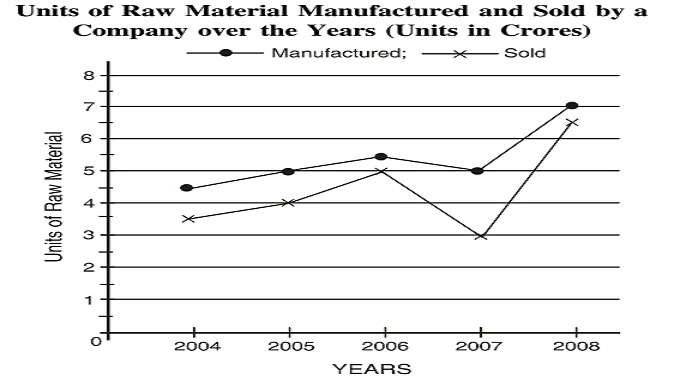Home » Data Interpretation » Line chart » Question

#### Data Interpretation

Direction: Study the following Graph carefully and answer the questions given below:1. What is the difference between the number of units manufactured and the number of units sold over the years?
1. 5 lakhs
2. 50 lakhs
3. 5 crores
4. 50 crores
##### Correct Option: C

Total number of units manufactured = Units manufactured in 2004 + Units manufactured in 2005 + Units manufactured in 2006 + Units manufactured in 2007 + Units manufactured in 2008
Total number of units manufactured = 4.5 + 5 + 5.5 + 5 + 7 = 27 crores
Total number of units sold = Units sold in 2004 + Units sold in 2005 + Units sold in 2006 + Units sold in 2007 + Units sold in 2008
Total number of units sold = 3.5 + 4 + 5 + 3 + 6.5 = 22 crores
Hence, their difference = 27 crores - 22 crores = 5 crores